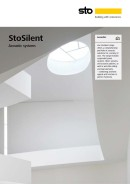Note: You are using Internet Explorer 11, which may have display errors, we recommend using a browser such as Mozilla Firefox, Google Chrome, Microsoft Edge or Safari

# Acoustic Systems

## Glossary

If you aren't familiar with acoustics, the terminology, standards and classes associated with the area can be a little confusing. This glossary explains some of the key terms you will encounter, from the basics of sound through to the many sound absorption classes used to categorise acoustic systems.

## Sound

An oscillation of pressure transmitted as a wave, and composed of frequency (Hz) and sound pressure level (dB).

Sound is perceived by the human ear in the decibel range of 0 to 120 dB and the frequency range of 20 to 20,000 Hz.

## Frequency (Hz)

The number of cycles per second of a sound wave, measured in Hertz (Hz). Frequency is perceived as sound pitch, with higher frequencies as higher pitched sounds.

The average adult human can perceive frequencies between 20 and 16,000 Hz, while young children can perceive frequencies up to 20,000 Hz.

## Sound Pressure Level (dB)

The pressure of a sound, measured in decibels (dB). Sound pressure is perceived as loudness. 0 dB is known as the threshold of human hearing, while 120 dB is known as the pain threshold.

## Sound Insulation (Dn,c,w)

The ability of a building element to reduce sound transmission through it, typically measured at frequencies of 100-4000 Hz.

Airborne sound insulation is expressed by the value Dn,c,w in accordance with ISO 140-9.

## Reverberation Time (RT60)

The time required, in seconds, for the average sound in a room to decrease by 60 dB after the source stops emitting sound. Reverberation Time is affected by the size of the space and the amount of reflective or absorptive surfaces within that space.

Highly sound absorbent surfaces will prevent it from reflecting back into the space, providing shorter reverberation times. Reflective surfaces will reflect the sound and increase the reverberation time within the space.

Larger spaces generally have longer reverberation times than smaller spaces and require more sound absorbent materials to achieve the same reverberation time as a smaller space.

## Speech Intelligibility

The clarity of speech in a room is dependent on the level of background noise, reverberation time and room shape.

Speech intelligibility is typically measured in rooms using the Rapid Speech Transmission Index (RASTI). A transmitter broadcasts a modulated noise signal from a loudspeaker, and a microphone receiver measures the clarity of the noise at the receiver position and gives a read-out of the RASTI value.

## Sound absorption (α)

The sound absorption properties of a material are expressed as a sound absorption coefficient α at specific frequencies. The α value is a ratio between 1 (all sound absorbed) and 0 (no sound absorbed).

## Sound absorption coefficient (αs)

The sound absorption coefficient at 1/3 octave band centre frequencies, measured in accordance with ISO 354. The results provide the most detailed values for sound absorption and form the basis for the simplified sound absorption classifications.

## Practical sound absorption coefficient (αp)

The average of the three αs values centred on the 1/3 octave band centre frequency, measured in accordance with EN ISO 11654.

A simplified yet accurate measurement of the sound absorption coefficient for practical use.

## Weighted sound absorption coefficient (αw)

Measured in accordance with ISO 11654. Practical sound absorption coefficient αp values at given standard frequencies are compared with reference curve αw.

The reference curve is shifted downwards in increments of 0.05 to the point where the sum of the negative deviations from the measured values is ≤ 0.10. The αw value is then recorded as the value of the reference curve at 500 Hz.

αw has been adopted as the European standard measurement for suspended ceilings.

## Sound Absorption Class A-E

Weighted sound absorption coefficient αw values are compared to a series of fixed reference curves, providing a general indication of the sound absorption capability of the material.

Absorption Classes A-E are described in international standard EN ISO 11654.

## Noise Reduction Coefficient (NRC)

A single value for sound absorption calculated in accordance with ASTM C423. The NRC is the mean average αs value at frequencies 250, 500, 1000 and 2000 Hz.

## Sabin

A unit of sound absorption. One square metre of 100% absorbing material has a value of one metric Sabin. The unit is named in honour of Wallace Clement Sabine.

The total absorption in sabins can be calculated by:

A = S1α1 + S2α2 + … + Snαn = ΣSiαi

A = the absorption of the room (m² sabin)

Sn = area of the actual surface (m²)

αn = absorption coefficient of the actual surface